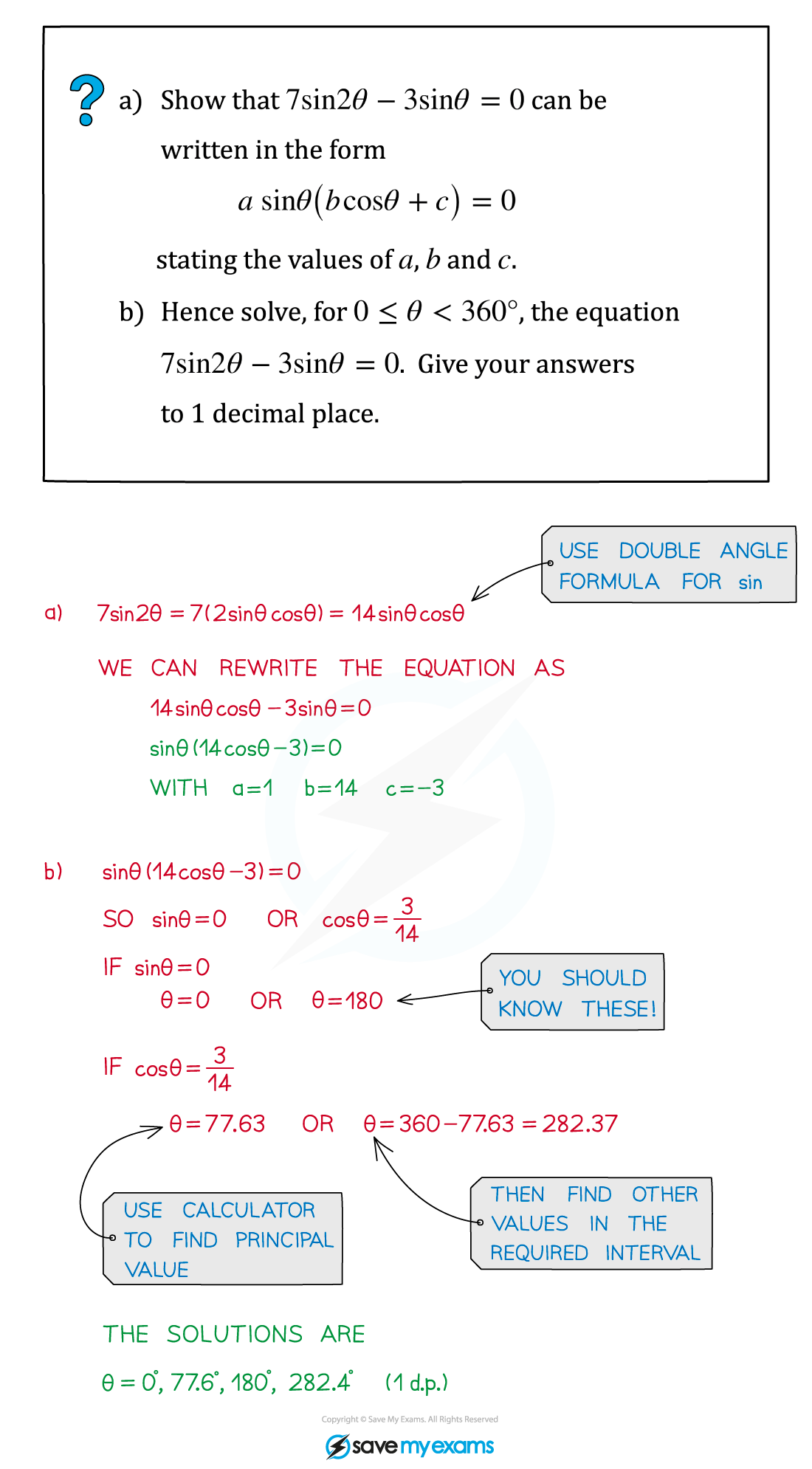# AQA A Level Maths: Pure复习笔记5.6.2 Double Angle Formulae

### Double Angle Formulae

#### What are the double angle formulae?

• The double angle formulae are derived from the 'A+B' versions of the compound angle formulae: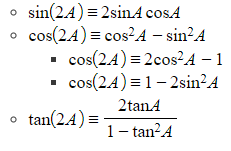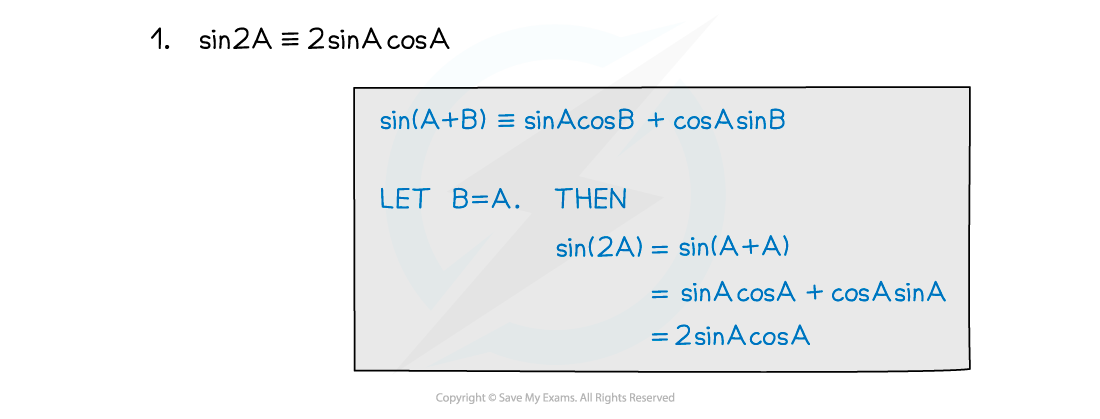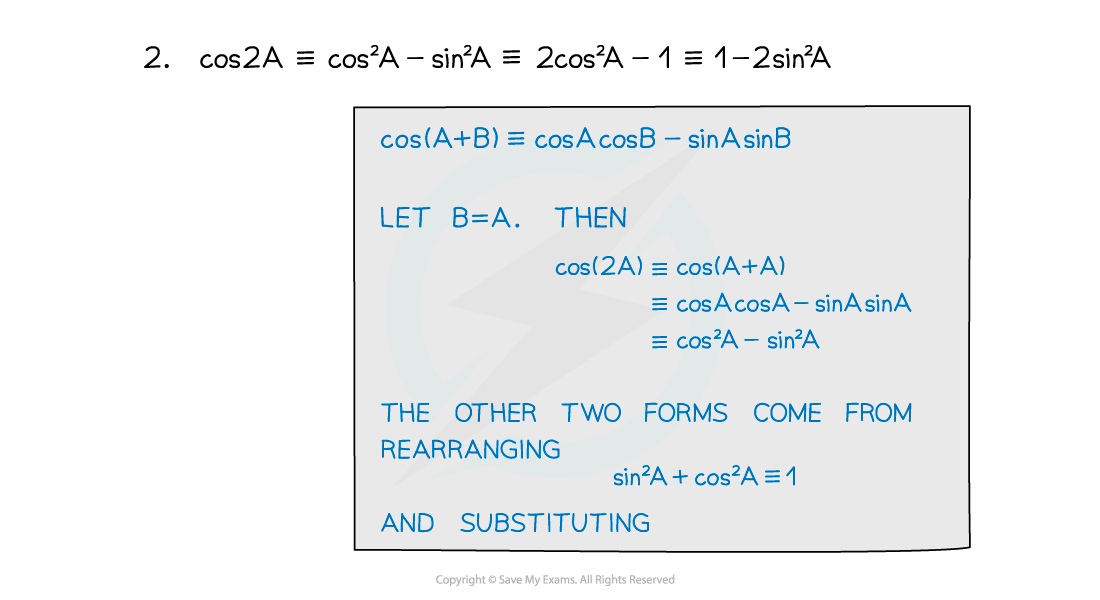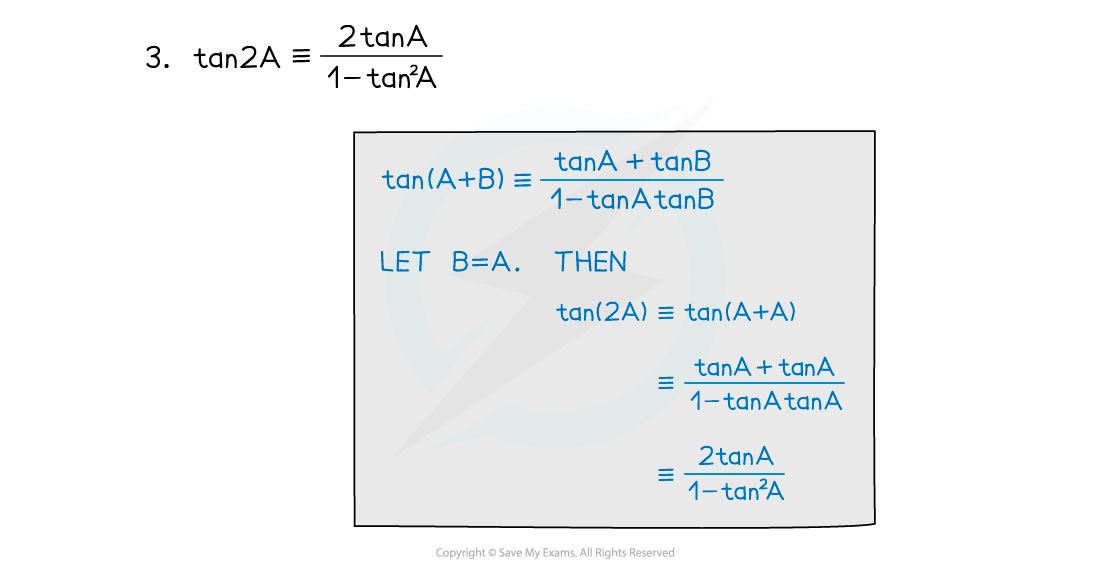#### Exam Tip

• The double angle formulae are not included in the formulae booklet – you have to know them.
• If you forget them, you can always derive them from the compound angle formulae (which are in the formula booklet) as shown above.

#### Worked Example